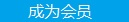您可以捐助，支持我们的公益事业。 1元 10元 50元 认证码：必填求知 文章 文库 Lib 视频 iProcess 课程 认证 咨询 工具 讲座 Modeler Code要资料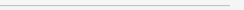订阅捐助
《深入浅出机器学习》之强化学习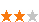818 次浏览     评价： 好 中 差
2018-11-21

 编辑推荐: 本文来自于简书，本文章主要通过举例来论证机器学习算法，通过矩阵进行强化学习介绍。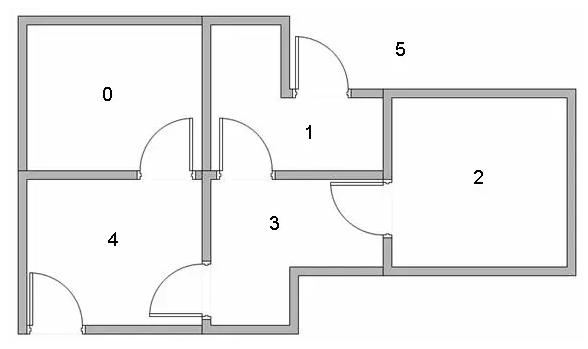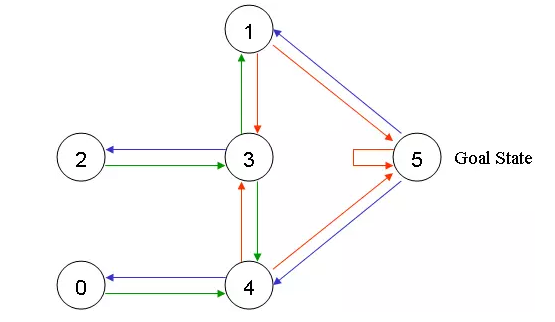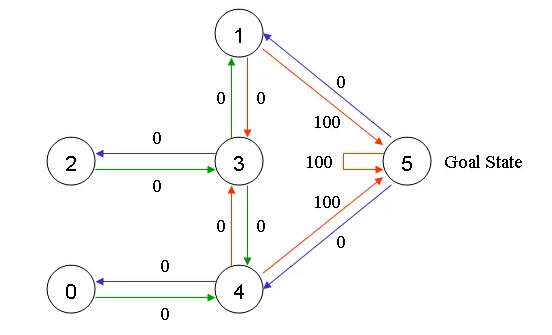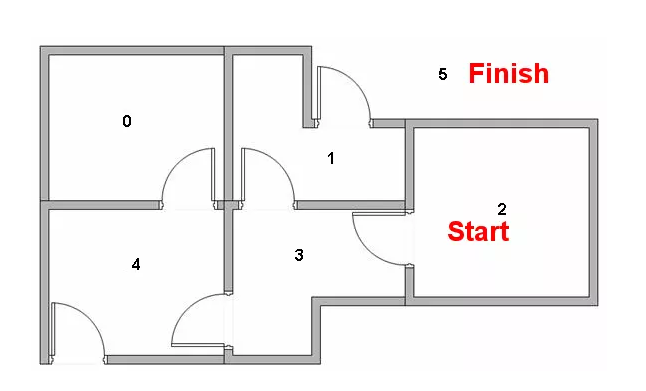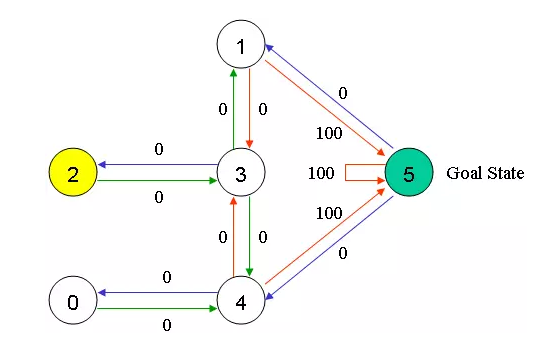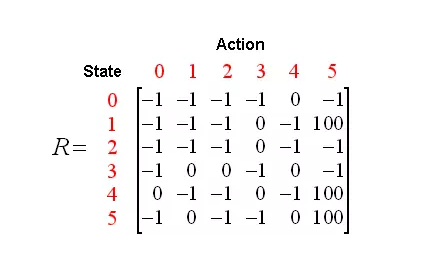Q矩阵初始化的时候全为0，因为它的状态我们已经全部知道了，所以我们知道总的状态是6。如果我们并不知道有多少个状态，那么请从1个状态开始，一旦发现新的状态，那么为这个矩阵添加上新的行和列。

Q(state, action) = R(state, action) + Gamma * Max[Q(next state, all actions)]

Q-learning算法如下：

1 设置gamma相关系数，以及奖励矩阵R

2 将Q矩阵初始化为全0

3 For each episode：

Do While 当没有到达目标时?

End Do

End For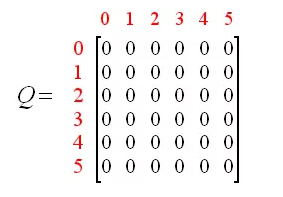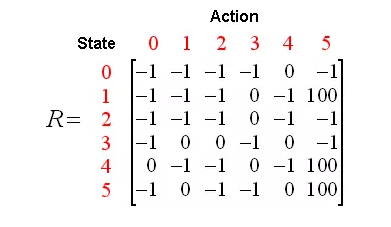Q(state, action) = R(state, action) + Gamma * Max[Q(next state, all actions)]

Q(1, 5) = R(1, 5) + 0.8 * Max[Q(5, 1), Q(5, 4), Q(5, 5)]= 100 + 0.8 * 0 = 100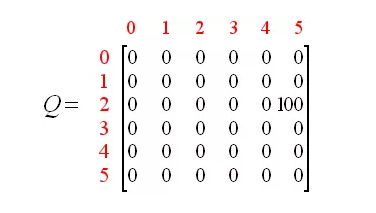Q(state, action) = R(state, action) + Gamma * Max[Q(next state, all actions)]

Q(3, 1) = R(3, 1) + 0.8 * Max[Q(1, 2), Q(1, 5)]= 0 + 0.8 * Max(0, 100) = 80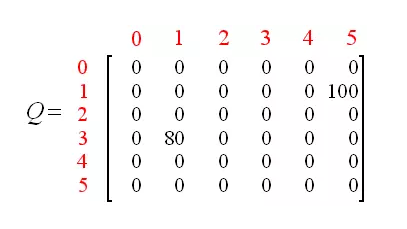Q(state, action) = R(state, action) + Gamma * Max[Q(next state, all actions)]

Q(1, 5) = R(1, 5) + 0.8 * Max[Q(1, 2), Q(1, 5)]= 0 + 0.8 * Max(0, 100) = 80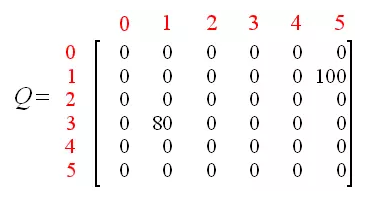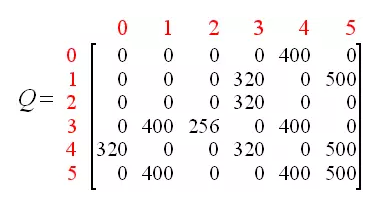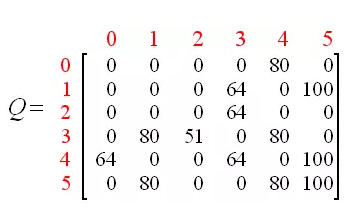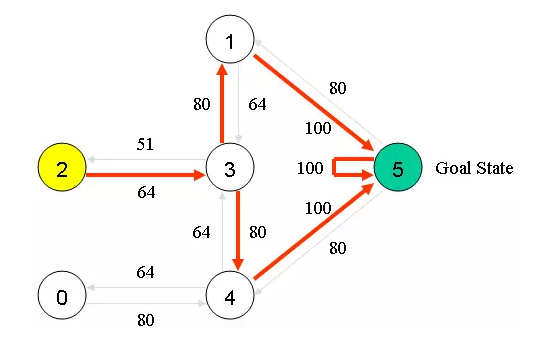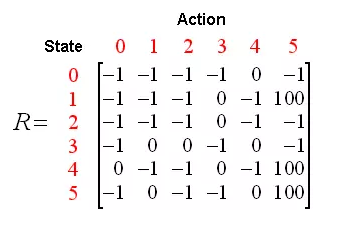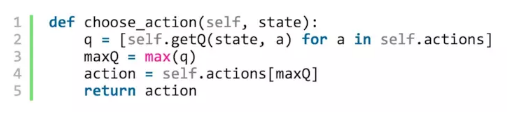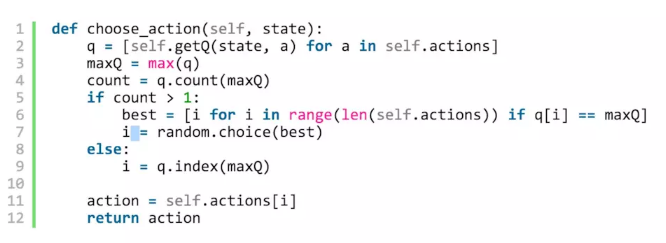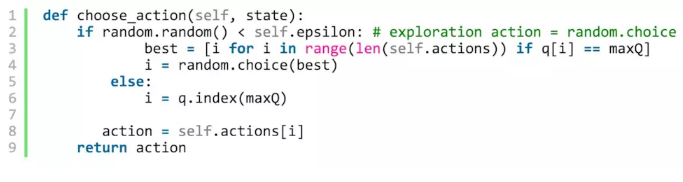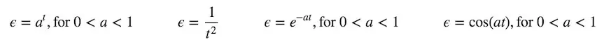818 次浏览  评价: 好 中 差订阅捐助
 相关文章 我们该如何设计数据库 数据库设计经验谈 数据库设计过程 数据库编程总结
 相关文档 数据库性能调优技巧 数据库性能调整 数据库性能优化讲座 数据库系统性能调优系列
 相关课程 高性能数据库设计与优化 高级数据库架构师 数据仓库和数据挖掘技术 Hadoop原理、部署与性能调优每天2个文档/视频 扫描微信二维码订阅
 订阅技术月刊 获得每月300个技术资源